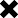### Regarding SIP + FV Calculator

POSTED BY ON June 26, 2012 5:50 pm COMMENTS (3)

HI,
I tried all the ways to get the figure which is mentioned below the calculator.
Example : Suppose you want to invest your money from total 30 yrs , out of which you want to pay from your pocket for 10 yrs and let that money grow for next 20 yrs . Then If you have invested 1,000 monthly for 10 yrs @12% , it will grow to 2,32,339 in 10 yrs , then once you leave that money for next 20 yrs @12% it will amount to 22,41,210 .
I gave my inputs as below:
Monthly Investment 1000
Payment Tenure 10
Return (Payment Tenure) 12
Investment Tenure (total Tenure) 30
Return (Investment Tenure) 20
I get the Payment Corpus : 232339 and Total amount Paid : 120000 as correct but could not get the figure “22,41,210” which is mentioned below the calculator.
Could you please throw some light in understanding that.
https://www.jagoinvestor.com/calculators/html/SIP-Future-Value-Calculator.html
Thanks

## 3 replies on this article “Regarding SIP + FV Calculator”

once you get the corpus =232339 at the end of 10 yrs , not that is the base capital and its earning 12% each year . If you apply simple compounded interest formula A * (1+r)^n

You will get

=232339*(1.12^20) = 2241210

1. Dear Madhava Ram, the calculator is ok. Please put nos. in following order.

Mly investment = 1000

Payment Tenure = 10 (years)

Return (Payment Tenure) = 12 (it’s actually the %age rate of return)

Investment Tenure = 30 (the total term of investment in years)

Return (Investment Tenure) = 12 (it’s actually the %age rate of return)

Thanks

Ashal

This site uses Akismet to reduce spam. Learn how your comment data is processed.